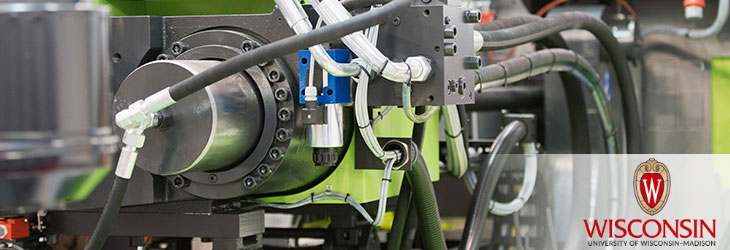Wisconsin Alumni Research Foundation

# WARF TECHNOLOGIES

EngineeringController For Power Converter
WARF: P140161US01

Inventors: Robert Lorenz, Yukai Wang, Takumi Ito

The Invention
A controller for a power converter detects a current output from the power converter, estimates a flux vector of the motor, and calculates a torque line in a physical model resulting from mathematizing a circuit equivalent to the motor, based on the estimated flux vector. The torque line indicates a line for obtaining a desired torque in a subsequent control period. The controller calculates a stator flux command value in accordance with a loss, calculates a voltage command value based on the torque line and the stator flux command value, and controls an output voltage.
Tech Fields